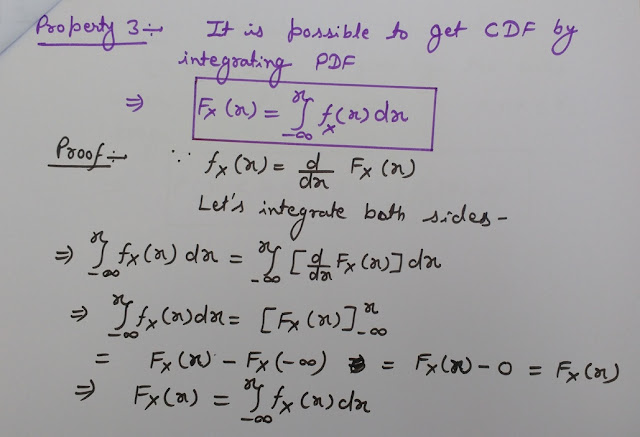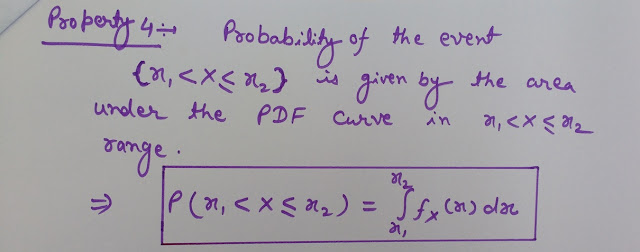## What is Probability Density Function (PDF)?

The derivative of Cumulative Distribution Function (CDF) w.r.t. some dummy variable is called as probability density function (PDF).
Probability density function can be defined mathematically as-Relation between PDF and CDF (Formula of PDF)

Now we will discuss the properties of probability density function. The derivation of properties of PDF is also provided here.

Cumulative Distribution Function (CDF) - Properties of CDF - CDF Definition, Basics - Continuous and Discrete CDF

## Properties of Probability Density Function (PDF) with Derivation

Property 1- Probability density function is always non-zero for all values of x.PDF Property 1 with Proof

Property 2- The area under the PDF curve is always equal to Unity i.e. one.PDF Property 2 With Proof

Joint Probability Density Function (Joint PDF) - Properties of Joint PDF with Derivation- Relation Between Probability and Joint PDF

Property 3- It is possible to get Cumulative Distribution Function (CDF) by integrating PDF.PDF Property 3 With Proof

Property 4- Probability of the event {x1< X<= x2} is given by the area under the PDF curve in
{x1< X<= x2} range.PDF Property 4 With Proof